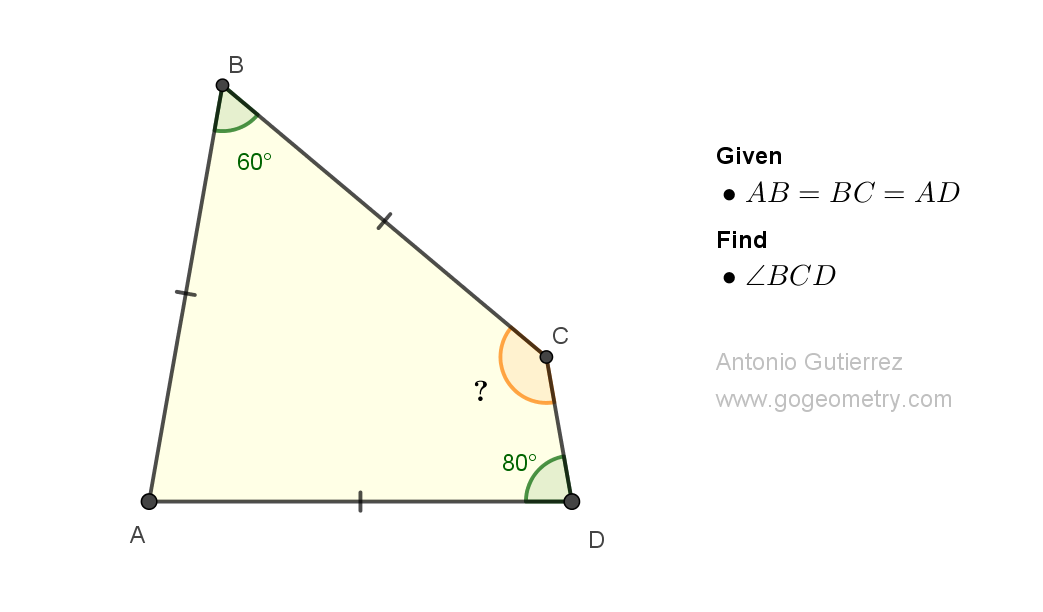## Sunday, February 19, 2023

### Geometry Problem 1508: Understanding Triangle Geometry: Isosceles, Equilateral, Quadrilateral, Angular. Difficulty Level: High School.

Geometry Problem 1508. Post your solution in the comment box below.
Level: Mathematics Education, K-12 School, Honors Geometry, College.

Details: Click on the figure below.More Details

To post a solution to this problem click Comment underneath the post, or click into the line that says, “Enter Comment.” Type what you want to say and press Publish to post your solution.

1.Note that triangle ABC is equilateral and triangle ACD is isosceles. So angle BCD=120 degrees

1.2.Yes Antonio. I made a mistake angle BCD= 60+80= 140

2.Triangle ABC is equilateral and Triangle ACD is thus isosceles (80-20-80)

Hence < C = 60 + 80 = 140

Sumith Peiris
Moratuwa
Sri Lanka

1.Some interesting results

1) If BC and AD meet at X, then Tr. CDX is isoceles (40-40-100)
2) If AB, DC meet at Y then Tr. AYD is isoceles 80-20-80
3) The diagonals of ABCD cut at an angle of 70